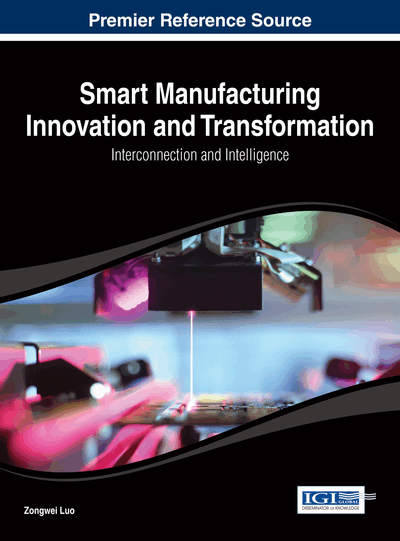# Meta-Heuristic Structure for Multiobjective Optimization Case Study: Green Sand Mould System

T. Ganesan (Universiti Technologi PETRONAS, Malaysia), I. Elamvazuthi (Universiti Technologi PETRONAS, Malaysia), K. Z. KuShaari (Universiti Technologi PETRONAS, Malaysia) and P. Vasant (Universiti Technologi PETRONAS, Malaysia)
DOI: 10.4018/978-1-4666-5836-3.ch003

## Abstract

In engineering optimization, one often encounters scenarios that are multiobjective (MO) where each of the objectives covers different aspects of the problem. It is hence critical for the engineer to have multiple solution choices before selecting of the best solution. In this chapter, an approach that merges meta-heuristic algorithms with the weighted sum method is introduced. Analysis on the solution set produced by these algorithms is carried out using performance metrics. By these procedures, a novel chaos-based metaheuristic algorithm, the Chaotic Particle Swarm (Ch-PSO) is developed. This method is then used generate highly diverse and optimal solutions to the green sand mould system which is a real-world problem. Some comparative analyses are then carried out with the algorithms developed and employed in this work. Analysis on the performance as well as the quality of the solutions produced by the algorithms is presented in this chapter.
Chapter Preview
Top

## Introduction

Currently, a series of issues have been raised when dealing with systems involving multiobjective optimization (MO) in engineering (Eschenauer et al., 1990; Statnikov & Matusov, 1995). In industrial engineering, scenarios which are multiobjective in nature are often encountered (where each of the objectives portrays different aspects of the problem). It is essential for the engineer to have access to numerous solution choices before selecting of the best solution. Some known strategies in MO optimization are Strength Pareto Evolutionary Algorithm (SPEA) (Zitzler & Thiele, 1998), Non-dominated Sorting Genetic Algorithm II (NSGA-II) by Deb et al. (2002), Weighted Sum method (Fishburn, 1967; Triantaphyllou, 2000), Goal Programming (Luyben & Floudas, 1994) and Normal-Boundary Intersection method (NBI) (Das & Dennis, 1998). These methods use concepts of Pareto-optimality to trace the non-dominated solutions at the Pareto curve and objective functions aggregation (scalarization) to solve these MO problems.

In green sand mould systems, the quality of the product obtained from the moulding process is very dependent on the physical properties of the moulding sand (such as; hardness, permeability, green compression strength and bulk density). Faulty extent of the mentioned properties may result in casting defects such as; poor surface finish, blowholes, scabs, pinhole porosity, etc. Controllable variables such as; percentage of water, percentage of clay, grain fineness number and number of strokes heavily influence the physical properties of the moulded sand. Therefore, by classifying these parameters as the decision variables and the mould sand properties as the objective function, the MO optimization problem was formulated in Surekha et al. (2011). The purpose of this formulation is for the determination of the best controllable parameters for optimal final-product of the moulding process. A more rigorous study on the optimization and model development of mould systems can be seen in Sushil et al. (2010) and Rosenberg (1967).

This chapter aims to discuss the implementation of meta-heuristic techniques for the production of a solution set that dominantly approximate the Pareto frontier in the objective space. These techniques applied in conjunction with the weighted-sum method improve the dominance of the solution set during successive iterations. In addition, the characteristics (convergence, diversity) of the solutions with regard to their level of dominance were also measured using performance metrics. A suitable real-world case study for this framework is the green sand mould systems.

This chapter is organized as follows; Section 2 presents the metaheuristic algorithms employed while Section 3 provides details on the Chaotic PSO algorithm developed in this work. Section 4 discusses the measurement metrics followed by Section 5 which presents the problem formulation. The computational results are analyzed in Section 6 and the chapter ends with the Concluding Remarks in Section 7.

## Complete Chapter List

Search this Book:
Reset